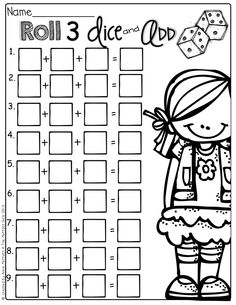# Probability 68564

What is the probability that the number
a) greater than 4,
b) the number greater than four will fall on the dice roll?

p1 =  0.3333
p2 =  0.6667

### Step-by-step explanation:Did you find an error or inaccuracy? Feel free to write us. Thank you!

Tips for related online calculators
Need help calculating sum, simplifying, or multiplying fractions? Try our fraction calculator.